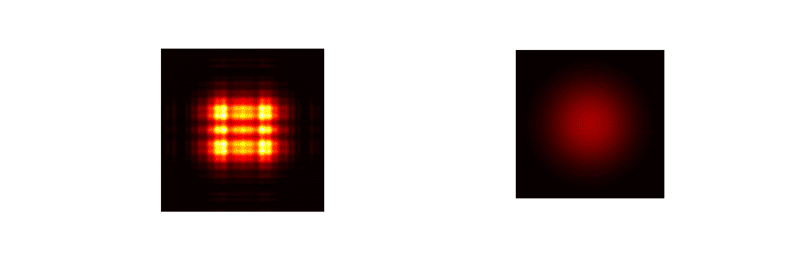# Intensity of a Gaussian laser beam

roam
I have two lasers with different intensity distributions (shown below) — one is Gaussian and the other one is rectangular (having the shape of a Fresnel diffraction pattern at the target).I am trying to compare the efficacy of the two lasers for burning a certain material (I am really comparing their wavelengths). But the two lasers have different total powers. I was told that the only way I can get a more direct comparison is to adjust the spot sizes so that the two have similar "intensities", i.e.,

$$\frac{P_{\text{Gaussian}}}{A_{\text{Gaussian}}}\approx\frac{P_{\text{Rectangular}}}{A_{\text{Rectangular}}}.$$

In many places, I have encountered people stating an intensity value in this way (dividing the total power of the beam by the beam cross-sectional area: ##I=P/A##). However, to me, it seems that this only works if you have a constant intensity distribution.

In fact, in optics textbooks, the intensity of a Gaussian beam is evaluated as the intensity of a single point within the x-y plane (power transferred per unit area):

$$I(x,y,z)=I_{0}\left[\frac{w_{0}}{w(z)}\right]^{2}\exp\left[-2\frac{\left(x^{2}+y^{2}\right)}{w(z)^{2}}\right].$$

where ##w(z)## is the radius of the beam at a distance ##z##. The concept seems to be only applicable to a given point — we can't really speak about the intensity of a beam spot as a whole. So does the usage of ##I=P/A## have any validity?

Is there any way I can get a more like-for-like comparison when the distributions of the lasers are different?

Any suggestions would be greatly appreciated.

#### Attachments

Homework Helper
Gold Member
The intensity (irradiance) is better described by ##\frac{dP}{dA} ##. In many cases, the almost parallel rays are brought to focus using an off-axis paraboloidal mirror. You can then get a diffraction limited spot, but if the source (e.g a HeNe) is close to the focusing mirror, instead of getting focusing in the focal plane, the lensmakers' equation ## \frac{1}{f}=\frac{1}{b}+ \frac{1}{m} ## will apply to determine where the beam gets focused=(at distance ## m ##). You might be interested in an Insights article I authored to get an understanding of the idea of diffraction-limited spot size: https://www.physicsforums.com/insights/diffraction-limited-spot-size-perfect-focusing/ ## \\ ## Less than perfect optics and less than perfect beam collimation will result in a spot size that is somewhat larger than the diffraction limit. You can also use a lens or spherical mirror to focus the beam, but the focused spot size will necessarily be larger than the diffraction limit. ## \\ ## And the intensity (irradiance watts/m^2) will not be uniform across the focused spot, but will normally peak in the center and fall-off in a manner similar to the far-field pattern. There are also some finer details about whether the focusing is in the focal plane, or at a distance ##m ## or at a location that is called the beam "waist". A google of the topic "beam waist" might show some of these results.

Last edited:
roam
I have read your article last year but my question is not really about tight focusing or the effects of focusing itself. We can suppose that the beam comes straight out of the laser and is not being focused down by lenses.

A lot of people divide the total power by the area of the beam to get what they variously call intensity or power density. So for a Gaussian beam with a radius ##w(z)## at the point ##z## where the measurement is taken, we have:

$$\frac{P_{\text{Total}}}{\pi w(z)^{2}}$$

What do you think about this quantity? Does this make sense given that the distribution of power is not uniform?Homework Helper
Gold Member
I have read your article last year but my question is not really about tight focusing or the effects of focusing itself. We can suppose that the beam comes straight out of the laser and is not being focused down by lenses.

A lot of people divide the total power by the area of the beam to get what they variously call intensity or power density. So for a Gaussian beam with a radius ##w(z)## at the point ##z## where the measurement is taken, we have:

$$\frac{P_{\text{Total}}}{\pi w(z)^{2}}$$

What do you think about this quantity? Does this make sense given that the distribution of power is not uniform?It does not give the exact irradiance at an arbitrary location in the beam, but without any beam profile information, it is a reasonably good number.

•roam
Cutter Ketch
So does the usage of I=P/AI=P/AI=P/A have any validity?

The usefulness and validity of using the local intensity compared to using the average over the area depends on the question you are asking. In regards to laser damage, it depends on how the energy is coupled into the material and how quickly the material dissipates the energy.

Not always, but usually, the localized intensity determines the lowest energy at which damage occurs (threshold). However the averaged energy determines how much damage is done. (Integrated energy).

•roam
Is there any way I can get a more like-for-like comparison when the distributions of the lasers are different?

Any suggestions would be greatly appreciated.

One possible metric is the so-called 'M2 value', which parametrizes how closely a laser beam approximates a Gaussian beam.

https://en.wikipedia.org/wiki/M_squared

However, the metric you really care about, 'I am trying to compare the efficacy of the two lasers for burning a certain material', may not be simply related to M2. A metric you may be more interested in is simply the peak irradiance- the maximum amount of power/area incident on the sample.

•roam